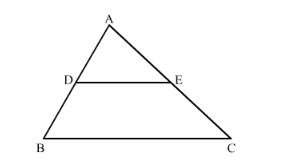# In the figure given below DE || BC. If AD = 2.4 cm,

Question:

In the figure given below DE || BC. If AD = 2.4 cm, DB = 3.6 cm, AC = 5 cm. Find AE.

Solution:

GIVEN: AD = 2.4cm, BD = 3.6cm and AC = 5cm.

TO FIND: AE

According to BASIC PROPORTIONALITY THEOREM If a line is drawn parallel to one side of a triangle intersecting the other two sides, then it divides the two sides in the same ratio.In ∆ABC, DE || BC.

$\frac{\mathrm{AD}}{\mathrm{AB}}=\frac{\mathrm{AE}}{\mathrm{AC}}$

$\frac{2.4}{2.4+3.6}=\frac{\mathrm{AE}}{\mathrm{AC}}$

$\frac{2.4}{6}=\frac{\mathrm{AE}}{5}$

$\mathrm{AE}=2$

$\mathrm{AE}=2 \mathrm{~cm}$